Find intervals of increasing/decreasing

Chapter 6 Class 12 Application of Derivatives
Concept wise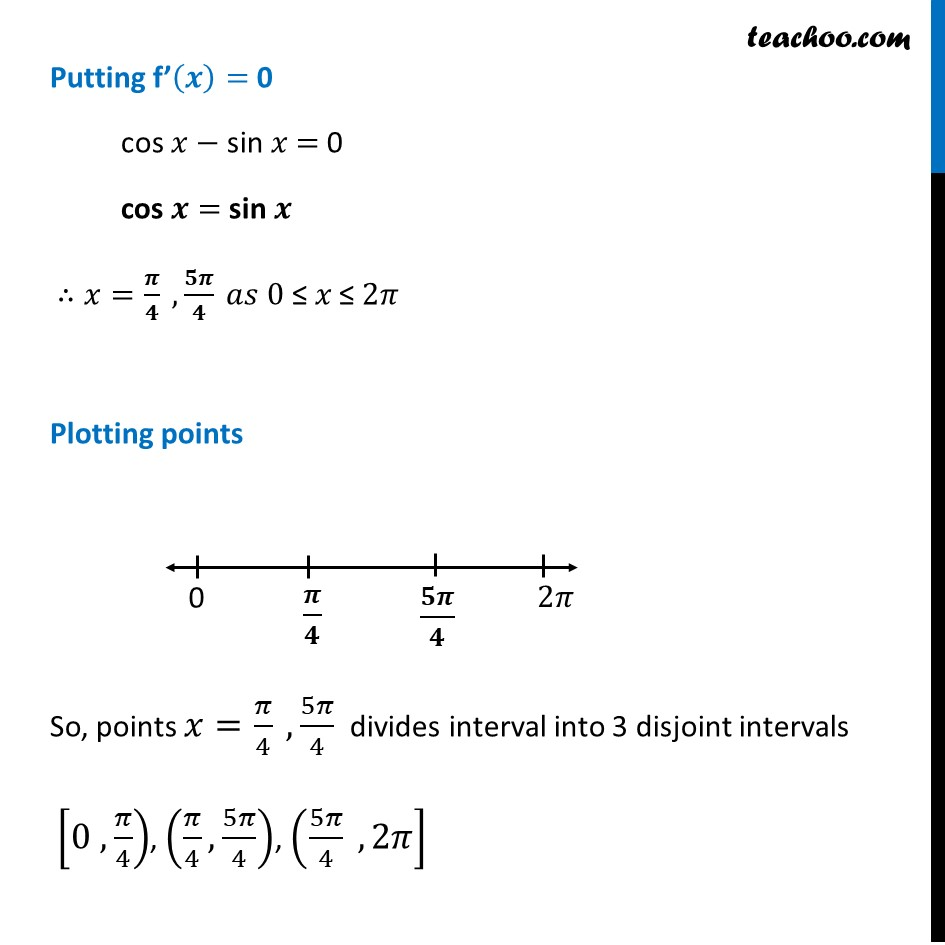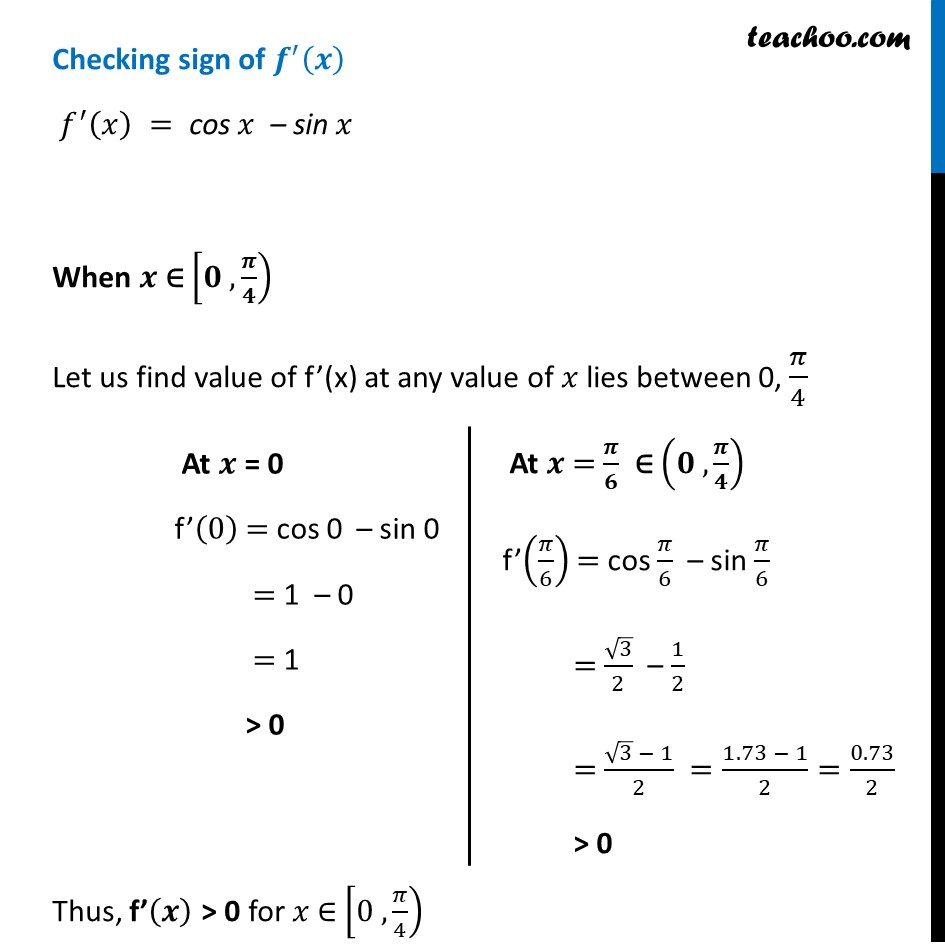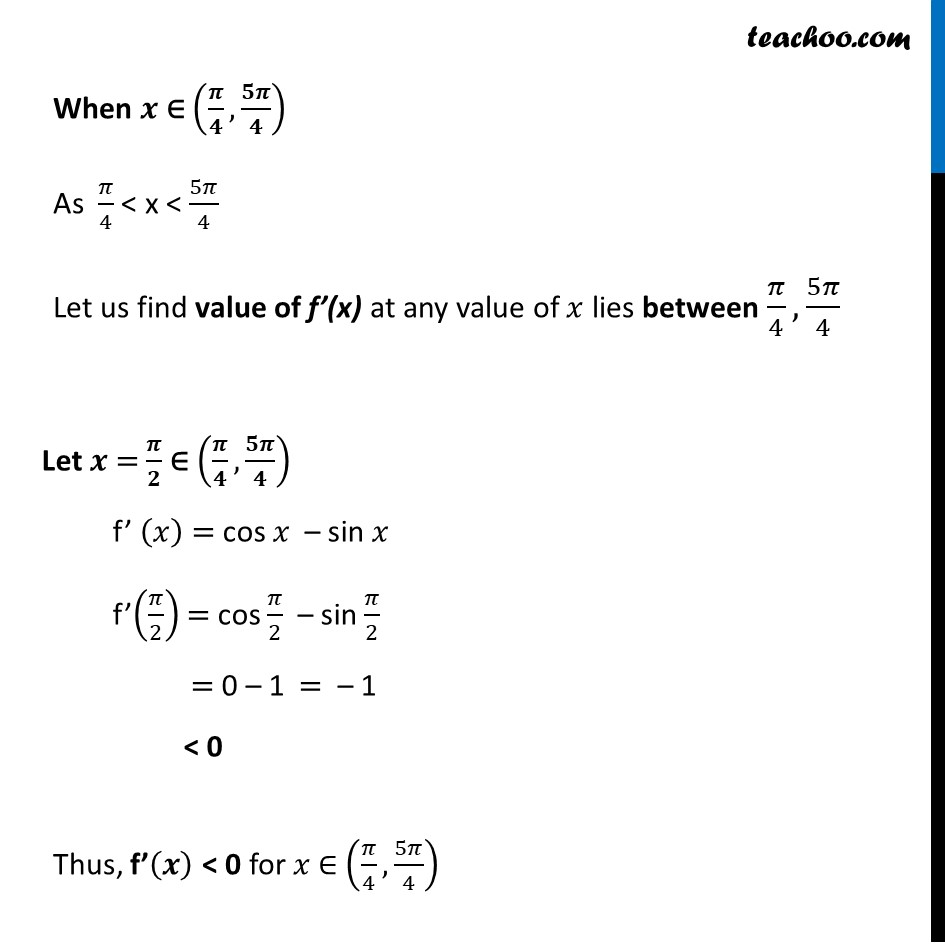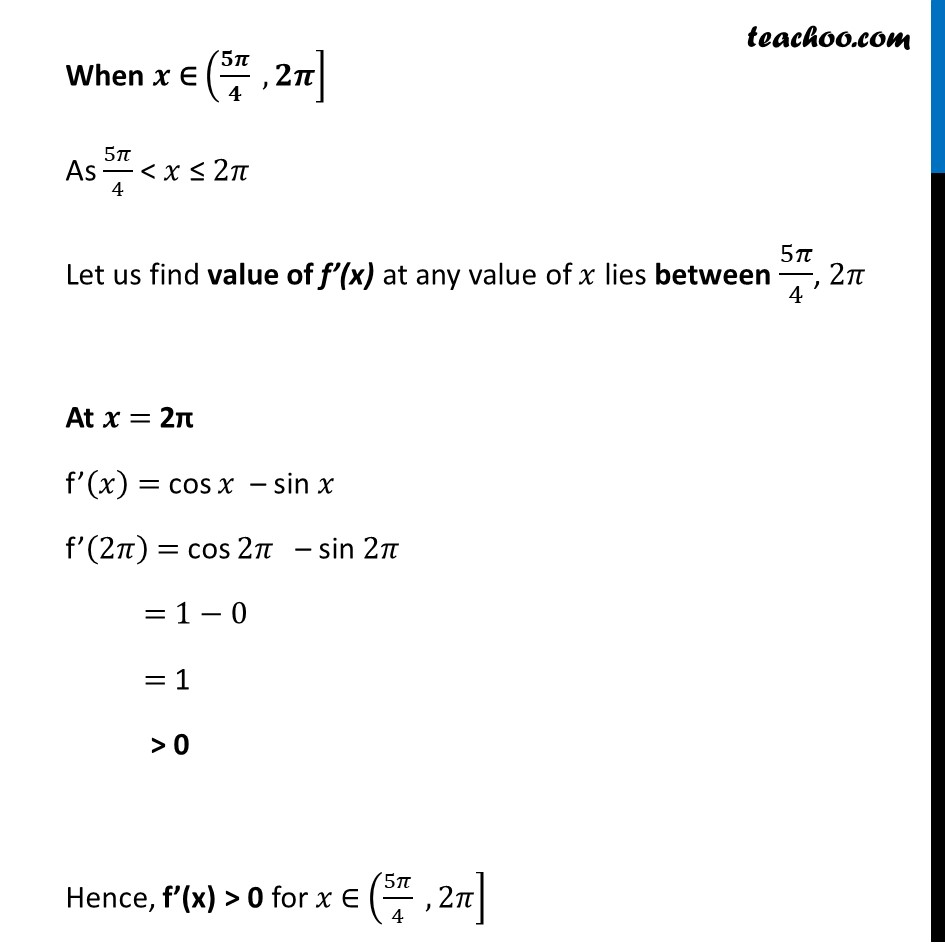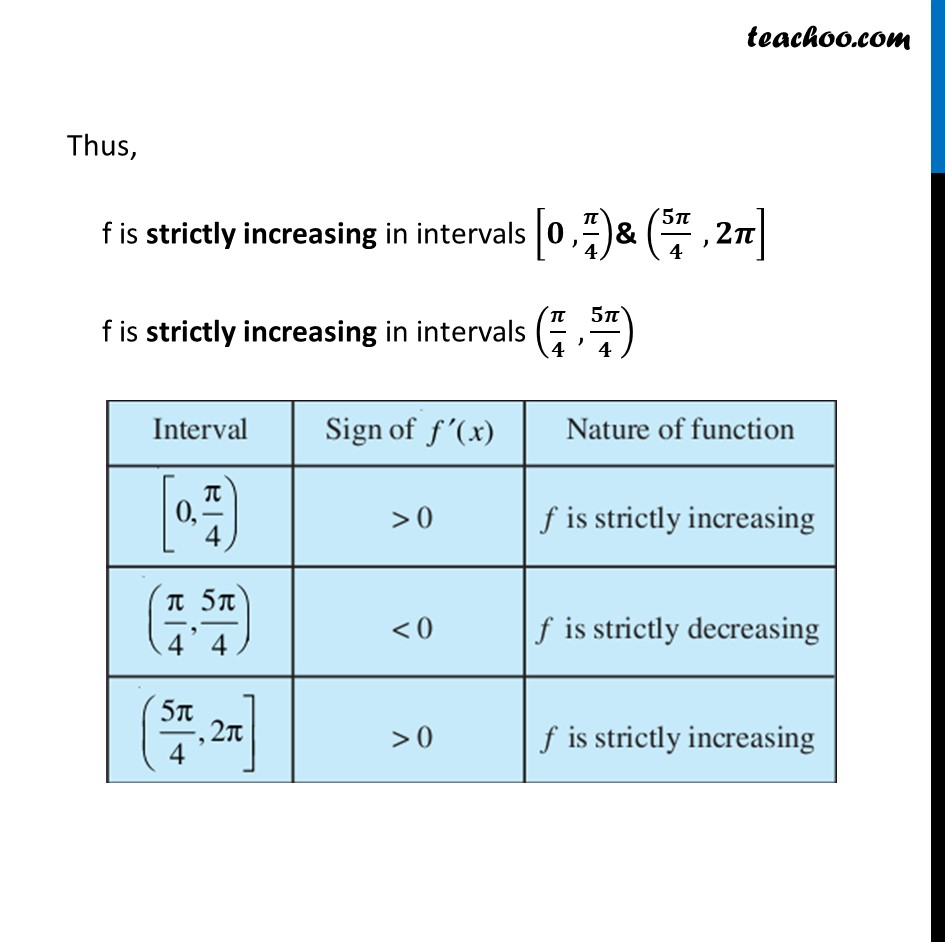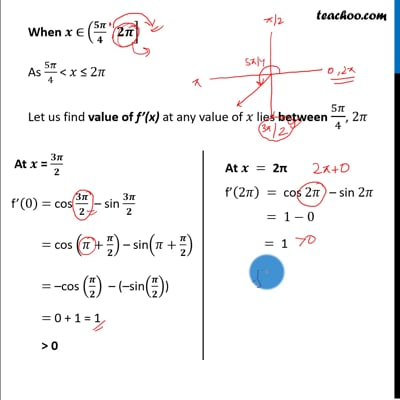This video is only available for Teachoo black users

Introducing your new favourite teacher - Teachoo Black, at only ₹83 per month

### Transcript

Example 13 Find the intervals in which the function f given by f (𝑥)=sin⁡𝑥+cos⁡𝑥 , 0 ≤ 𝑥 ≤ 2𝜋 is strictly increasing or strictly decreasing.f(𝑥) = sin 𝑥 + cos 𝑥 Finding f’(𝒙) f’(𝑥) = (𝑑 )/𝑑𝑥 (sin 𝑥 + cos 𝑥) f’(𝑥) = 𝑑(sin⁡𝑥 )/𝑑𝑥 + 𝑑(cos⁡𝑥 )/𝑑𝑥 f’(𝑥) = "cos " 𝑥 + (−𝑠𝑖𝑛𝑥) f’(𝒙) = 𝒄𝒐𝒔⁡𝒙 – 𝒔𝒊𝒏⁡𝒙 Putting f’(𝒙) = 0 cos 𝑥 − sin 𝑥 = 0 cos 𝒙 = sin 𝒙 ∴𝑥=𝝅/𝟒 ,𝟓𝝅/𝟒 𝑎𝑠 0" ≤ " 𝑥 ≤ 2𝜋 Plotting points So, points 𝑥=𝜋/4 ,5𝜋/4 divides interval into 3 disjoint intervals [0 , 𝜋/4), (𝜋/4,5𝜋/4), (5𝜋/4 , 2𝜋] Checking sign of 𝒇^′ (𝒙) 𝑓^′ (𝑥)" "=" cos " 𝑥" – sin " 𝑥 When 𝒙 ∈ [𝟎 , 𝝅/𝟒) Let us find value of f’(x) at any value of 𝑥 lies between 0, 𝜋/4 Thus, f’(𝒙) > 0 for 𝑥 ∈ [0 , 𝜋/4) At 𝒙 = 0 f’(0) = cos 0 – sin 0 = 1 – 0 = 1 > 0 At 𝒙 = 𝝅/𝟔 ∈ (𝟎 , 𝝅/𝟒) f’(𝜋/6) = cos 𝜋/6 – sin 𝜋/6 = √3/2 – 1/2 = (√3 − 1)/2 =(1.73 − 1)/2=0.73/2 > 0 When 𝒙 ∈ (𝝅/𝟒,𝟓𝝅/𝟒) As 𝜋/4 < x < 5𝜋/4 Let us find value of f’(x) at any value of 𝑥 lies between 𝜋/4, 5𝜋/4 Thus, f’(𝒙) < 0 for 𝑥 ∈ (𝜋/4,5𝜋/4) Let 𝒙 = 𝝅/𝟐 ∈ (𝝅/𝟒,𝟓𝝅/𝟒) f’ (𝑥) = cos 𝑥 – sin 𝑥 f’(𝜋/2) = cos 𝜋/2 – sin 𝜋/2 = 0 – 1 = – 1 < 0 When 𝒙 ∈ (𝟓𝝅/𝟒 , 𝟐𝝅] As 5𝜋/4 < 𝑥 ≤ 2𝜋 Let us find value of f’(x) at any value of 𝑥 lies between 5𝜋/4, 2𝜋 At 𝒙 = 2π f’(𝑥) = cos 𝑥 – sin 𝑥 f’(2𝜋) = cos 2𝜋 – sin 2𝜋 = 1−0 = 1 > 0 Hence, f’(x) > 0 for 𝑥 ∈ (5𝜋/4 , 2𝜋] When 𝒙 ∈ (𝟓𝝅/𝟒 , 𝟐𝝅] As 5𝜋/4 < 𝑥 ≤ 2𝜋 Let us find value of f’(x) at any value of 𝑥 lies between 5𝜋/4, 2𝜋 At 𝒙 = 2π f’(𝑥) = cos 𝑥 – sin 𝑥 f’(2𝜋) = cos 2𝜋 – sin 2𝜋 = 1−0 = 1 > 0 Hence, f’(x) > 0 for 𝑥 ∈ (5𝜋/4 , 2𝜋] Thus, f is strictly increasing in intervals [𝟎 , 𝝅/𝟒)& (𝟓𝝅/𝟒 , 𝟐𝝅] f is strictly increasing in intervals (𝝅/𝟒 , 𝟓𝝅/𝟒)